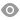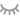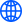中文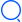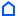SCARA 机器人运动会第二场：搬运速度和止振时间大比拼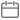2021-10-29 14:18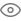71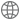# SCARA 机器人运动会第二场：搬运速度和止振时间大比拼

SCARA 机器人运动会在2021年的夏秋之际拉开帷幕！数位代表着行业内顶尖水准的国内外选手精心准备十余载，只为一朝惊艳世人。（标准节拍大比拼视频）

vs

（李群止振）                                                                         （邻国选手止振）

（大回环视频比拼视频）P = (0,0)

P = (90,0)

P = (115,0)

P = (130,85)

P = (75,135)

P = (-50,135)

P = (50,-135)

P = (-75,-135)

P = (-130,-85)

P = (-115,0,40)

P = (-90,0)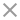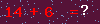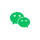微信扫码You are not yet a member Register nowregisterYou are not yet a member Register nowEmail registration